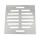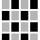# Windows

Calculate the area of masonry to build wall with dimensions of 9 m × 4 m with 4 windows of size 64 cm × 64 cm.

Result

S =  34.4 m2

#### Solution:Leave us a comment of example and its solution (i.e. if it is still somewhat unclear...):

Showing 0 comments:Be the first to comment!#### To solve this verbal math problem are needed these knowledge from mathematics:

Do you want to convert area units?

## Next similar examples:

1. Square plateFrom a square metal plate with a side long 6dm were cut two identical rectangular openings with dimensions of 2.5 dm and 2 dm. Calculate the remainder of the square plate.
2. Decorative fenceThe rectangular garden is 57 m long and 42 m wide. Calculate how much m² it will reduce if it encloses with a 60 cm wide decorative fence.
3. Area of rectangleCalculate the area of rectangle in square meters whose sides have dimensions a = 80dm and b = 160dm.
4. AreaCalculate: ?
5. SquaresCalculate the area of square and result round to square decimeters. a) a = 1,52 dm b) a = 13 268mm c) a = 562 cm d) a = 1,52 m
6. Area of squareCalculate the content area of the square whose perimeter is 24 dm.
7. Square glassWhat is the area of the square window glass with the side 4 dm? Do you know how many cm2 is it?
8. Argicultural fieldField has a rectangular shape with a width 180 m and circumference 940 m. How many hectares acreage has field?
9. Rectangle vs squareThe rectangle has dimensions of 13 × 10, square 8 × 8. Which shape has more area and how much above?
10. TailorTailor has an estimated 11 meters of 80 cm wide fabric. He will shape wholly 7 rectangles measuring 80 x 150 cm and nothing fabric does not remain. How many fabric he have?
11. LandLand has a rectangular shape, its surface area is 1.45 hectares. Its width is 250 m. Determine the length of the land.
12. RainfallHow many liters of water did fell in a 32m long and 8m wide garden, if 8mm of rain fell?
13. Bathroom 2A bathroom is 2.4 meters long and 1.8 meters wide. How many square tiles 1 dm on each side are to be used to cover it?
14. Rhombus sidesfind the sides of a rhombus, it area is 550 cm square and altitude is 55cm
15. One hectareHow many square meters are one hectare?
16. Addition of Roman numbersAdded together and write as decimal number: LXVII + MLXIV
17. ProductResult of the product of the numbers 1, 2, 3, 1, 2, 0 is: